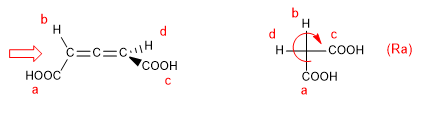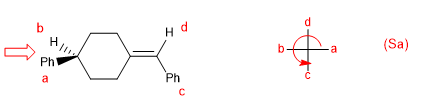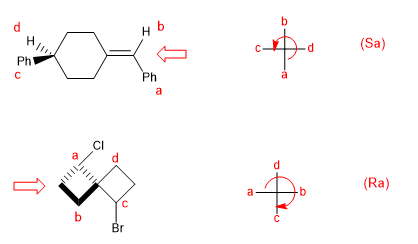In molecules whose chirality element is an axis, we will give the notation R a / S a , (the subscript "a" refers to axial), by means of the Fischer projection method. The tetrahedron method can also be used.1. For the molecule to have a chirality axis, it is necessary that the two groups on each side are different from each other. In this example the groups are different (-H and -COOH) and the molecule has a chirality axis.

2. We choose one side of the allene (I arbitrarily choose the left one) and begin to give priorities by atomic number to the substituents on that side. Then we move to the right side and give the "c,d" priorities to the groups on that side.3. Located where the red arrow is, we make the Fischer projection of the molecule4. We make the turn "a,b,c", if the rotation is clockwise the notation is Ra, if it is in the opposite direction the notation will be Sa. The position of the group d does not matter in this type of notation.Note that giving the priorities starting from the right hand side does not change the notation.In this last example we have given absolute notation to a spiro compound.
Two examples of biphenyls: